Students get through the MP Board Class 11th Chemistry Important Questions Chapter 3 Classification of Elements and Periodicity in Properties which are most likely to be asked in the exam.

## MP Board Class 11th Chapter 3 Classification of Elements and Periodicity in Properties

Classification of Elements and Periodicity in Properties Class 11 Important Questions Very Short Answer Type

Question 1.
What is Mendeleev’s periodic law?
The law on the basis of which Mendeleev classified the elements, is called Mendeleev’s periodic law. According to this, “physical and chemical properties of elements are periodic functions of their atomic weights.” i.e.,When elements are arranged in the increasing order of their atomic masses they show periodicity in their properties after a definite interval.

Question 2.
Define modern periodic law.
Modern periodic law : Physical and chemical properties of elements are periodic functions of their atomic numbers. On the basis of this law when elements are arranged in the increasing order of their atomic number they show a periodicity in properties after a definite interval.

Question 3.
Discuss the law on the basis of which Mendeleev classified the elements. What modifications are done in this law ?
The law on the basis of which Mendeleev classified the elements is known as Mendeleev’s Periodic law. According to this law “The physical and chemical properties of elements are a periodic function of their atomic mass”. Now this law is modified and is known as Modem Periodic law. According to this law “The physical and chemical properties of elements are a periodic function of their atomic number.”

Question 4.
What is Dobereiner’s law of Triad ?
This law was proposed by Dobereiner. According to this law, “Of the three similar atoms, the atomic mass of the middle element is the average mean of the other two elements.
Li7, Na23, K39,i.e $$\frac{39+7}{2}$$ = 23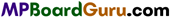Question 5.
On the basis of quantum numbers prove that there should be 32 elements in the sixth period of the periodic table.
In long form of periodic table each period starts with the filling of a new energy shell (n). For the sixth period n = 6. In this period, electrons enter in 6s,4f, 5d and 6p. Total 16(1+7 + 5 + 3) orbitals are there in these subshells. According to Pauli’s exclusion prin¬ciple, each orbital can have maximum two electrons. Thus, in 16 orbitals these can be 32 electrons. Therefore, sixth period should have 32 elements.

Question 6.
What is Newland octave rule?
Newland arrange the element in order of their atomic masses he found that the eighth element is a kind of repetition of first element, just like eighth note of music. This arrangement of element is known as Newland law of octave or Newland octave rule.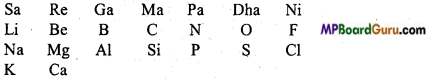Question 7.
State the period and group where Z = 114 will be situated?
Maximum value of n will be represented by the electronic configuration of the element.
114Z=86[Rn]7s2,5f14,6d10,7f2
Period = 7,
(∵ n = 7)
Group =14(10 + 2 + 2).

Question 8.
Write the atomic number of the element which is situated in the 3rd period and 17th group.
Outermost configuration of elements situated in 17th group is ns2 np2. In the third-period valence shell configuration of the element will be 3s2 3p5. In the 3rd period, atomic number starts from atomic number Z = 11 and ends in Z = 18. Thus, the given atomic number =10 + 2 + 5=17.

Question 9.
Name of which element is proposed by the following :
(i) By Lawrence Berkeley Laboratory,
(ii) By Sea borg’s group.
(i) Lawrencium (Z = 103) and Berkelium (Z = 97)
(ii) Seaborgium (Z = 106).

Question 10.
Why are the physical and chemical properties of the elements of a group same?
The outer electronic configuration of the elements of a group is same due to which they show similar physical and chemical properties.

Question 11.
Why are cations smaller than their parent atoms and anions larger than their parent atoms? Explain.
Cations are always smaller than their parent atoms because due to the removal of one or two electrons, magnitude of their effective nuclear charge increases. This results in greater attractive force of the nucleus on the valence electrons due to which ionic radius decreases.

Alternatively, anions are always larger than their parent atoms because due to the gain of one or two electrons magnitude of effective nuclear charge decreases by which attractive force of nucleus with the valence electron decreases. This results in decrease in ionic radius.

Question 12.
How many periods and groups are in Mendeleev’s periodic table? What is the number of elements in each period?
There are 7 periods and 9 groups in Mendeleev’s Periodic table. First period consists of 2 elements, second and third period consist of 8-8 elements, fourth and fifth period contain 18-18 elements and sixth period consist of 32 elements. Seventh period is incomplete. In it, elements from atomic number 90 to 103 called Actinides are also included.Question 13.
What changes occur on moving from left to right in every period?
In every period, on moving from left to right ionization potential and electron affinity increases but metallic nature, basicity of oxides and atomic radius decreases.

Question 14.
What changes occur on moving from top to bottom in a group?
On moving from top to bottom in a group atomic radius, ionic radius increases but ionization energy, electronegativity, electron affinity and melting point decreases.

Question 15.
Compare Modern periodic table with Mendeleev’s periodic table (any 3 points).
Comparison between Modern periodic table and Mendeleev’s periodic table

 Modern periodic table Mendeleev’s periodic table 1. In modern periodic table elements are arranged in the increasing are order of their atomic numbers. In Mendeleev’s periodic table elements arranged in the increasing order of their atomic masses. 2. In it, elements are divided in 18  groups. In it, elements are divided in 9 groups and subgroups. 3. In it, metals and non-metals are kept separately. In it, metals and non-metals are not kept separately.

Question 16.
What is diagonal relationship? Give an example.
Certain elements of the 2nd period show resemblance with some elements of the 3rd period. Thus Li resembles Mg, Be resembles Al, B resembles Si in certain respects.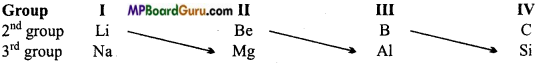Question 17.
What are the various factors due to which the ionization enthalpy of the main group elements tends to decrease down a group?
Decrease in ionization enthalpy down the group in the elements of main groups is due to the following two reasons :
(i) On moving down the group atomic size increases, due to increase in the formation of new energy shell due to which value of effective nuclear charge of the nucleus for the valence electron gradually decreases.

(ii) On moving down the group, screening effect increases, due to which ionization enthalpy decreases (Because attractive force between the nucleus and electron decreases).

Question 18.
What is the main difference between electron gain enthalpy and electronegativity?
Electron gain enthalpy is the nature of formation of a gaseous anion by gaining an electron by an isolated gaseous atom whereas electronegativity is the nature of an atom of an element to attract the shared pair of electrons present in a covalent bond towards itself. Like electron gain enthalpy, electronegativity also has no measuring unit.

Question 19.
In all nitrogen compounds, Electronegativity of N in Pauling scale is 3.0. What reaction will you give on this statement?
Electronegativity of N in all nitrogen compounds in Pauling scale is 3.0. This statement is incorrect because electronegativity of any element is not definite, the effect of the other element with which it is bonded is also applied on its value. Its value increases with the oxidation state of the element and with the increase in percent 5-character of the hybrid orbital.

Question 20.
Covalent radius: In homoatomic molecules containing same type of atoms bonded together by single covalent bond, covalent radius may be defined as, “one half of the distance between the centres of the nuclei of two adjacent similar atoms joined together by a single covalent bond”.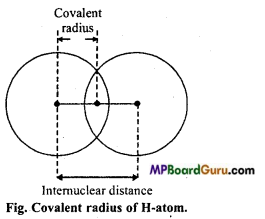Question 21.
What is van der Waals’ radius ?
van der Waals’ radius: It is defined as, “one half of the internuclear distance between two adjacent atoms belonging to two nearest neighbouring molecules of the substance in the solid-state”.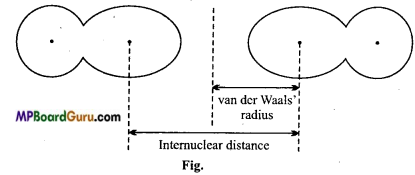Question 22.
What do you understand by normal elements?
Such elements which represent their group are called typical elements. Their only one outer shell is incomplete and all inner shells are completely filled according to the rules. Elements of second and third period are called typical elements.
Example: C, N, O etc.Question 23.
First ionisation enthalpy of two isotopes of an element will be same or different ? What do you believe ? Justify your answer.
First ionisation enthalpy of two isotopes of an element should be same because its value depends on electronic configuration and effective nuclear charge. Electronic configuration of the isotopes of an element is same due to which nuclear charge is also similar.

Question 24.
Write general outer electronic configuration of s-, p-, d- and f-block elements.
s- block elements : ns1-2 (n = 2-7)
p- block elements : ns2np1-6 (n = 2-7)
d- block elements : (n – l)d1-10ns0-2 (n = 3-7)
f-block elements : (n-2)f 1-14 (n-1)d,10ns2(n =6-7)

Question 25.
Ionization potential of Nitrogen is higher than oxygen. Explain.
Electronic configuration of nitrogen and oxygen is 7N = 1.s2, 2s2 2p3 (half filled 2p -orbitals) 8O = 1.s2, 2s2 2p4
Thus in case of nitrogen, 2p subshell is half-filled and removal of electron from half-filled orbitals or full-filled orbitals require more energy than that of other incomplete orbitals. So, ionization energy of nitrogen is greater than oxygen because of half filled p-subshell.

Question 26.
Ionisation potential of inert gases are very high. Why?
Electronic configuration of inert gases is most stable so it is very difficult to pull an electron. So in each period, ionisation potential of inert gases are very high.

Question 27.
Halogens have very high electron affinity. Why?
Halogens are one electron short to attain the stable electronic configuration of inert gases. So these elements have very high tendency to accept electron. Thus the electron affinity of halogens is very high.

Question 28.
Among F and Cl, whose electron affinity is higher and why?
Electron affinity of F is less than Cl because due to small size of F repulsion of its 2p electrons is more. Therefore added electron does not provide extra stability to the atom

Question 29.
Electron affinity of Noble Gases is zero. Why?
Electronic configuration of Noble gases is stable, because their valence shells are completely filled and all the electrons are paired, due to which there is no affinity for the incoming electron. Therefore, electron affinity of noble gases is zero.

Question 30.
Differences between Atomic and Ionic radius

 Atomic radius Ionic radius 1. Distance from the nucleus of a neutral atom to the outermost shell is called atomic radius. Distance from the nucleus to the outer- most shell of the ion formed from a neutral atom is called ionic radius. 2. Atomic radius is larger than the ionic radius of the cation. Radius of cation is smaller than the atomic radius but radius of anion is larger than the atomic radius.

Question 31.
Value of second ionization potential of an element is more than the value of first ionisation potential. Why?
By the removal of an electron from an atom, the remaining atom becomes posi¬tively charged. Ionic radius of cation is smaller than atomic radius, due to which more energy is required to remove the second electron from the outermost shell. Therefore, value of second ionisation potential is higher than first ionization potential.Question 32.
Ionic radius of cation is smaller than atomic radius. Why? Or, Size of cation is smaller than the corresponding atom. Why?
A cation is formed by the loss of one or more electrons from an atom. In this process, number of protons in the nucleus and nuclear charge present in the nucleus remains the same, but number of electrons present in the outermost shell decreases. As a result nuclear attractive force per electron increases and outer shell gets strongly attracted by the nucleus. Therefore, size of cation is smaller than the corresponding atom.

Question 33.
Ionic radius of anion is larger than the atomic radius. Why? Or, Size of anion is bigger than the corresponding atom. Why?
An anion is formed by the gain of one or more electrons by an atom. In this process, number of protons in the nucleus and nuclear charge present in the nucleus remains the same, but number of electrons present in outermost shell increases. As a result, nuclear attractive force per electron decreases due to which size of anion becomes larger than the corresponding atom.

Question 34.
Electron affinity of Be and N is almost zero. Why?
2s sub-shell of Be is more stable as it is fully filled and it does not allow any extra electron to enter into the s sub-shell. Similarly, p sub-shell of N is more stable due to half filled configuration. It does not gain any extra electron. Therefore, electron affinity of Be and N is almost zero.

Question 35.
Mg2+ion is smaller than O2- while electronic configuration of both are same. Why?
The nuclear charge on Mg2+ and O2- is +12 and +8 respectively but in both number of electrons is 10. Their electronic configuration is 1s2,2s2 2p6. In Mg2+ there is attraction between 10 electrons and 12 protons and in O2 –ion there is force of attraction
between 10 electrons and 8 protons and force of Mg+2 is greater than oxygen, so Mg+2 is smaller than O2- ion.

Question 36.
First ionization potential of A1 is less than Mg. Why?
Ans. Electronic configuration of Al and Mg is as follows :
Al13: 1s2, 2s22p6a, 3s23p1
Mg12 : 1s2, 2s22p6, 3s2,
Half filled or fully filled orbitals are more stable, p sub-shell of Al is incomplete whereas s sub-shell of Mg is completely filled. Thus, sub-shell of Al is less stable than sub shell of Mg. Therefore to remove an electron from Al atom less energy is required as compared to Mg. Therefore, ionization energy of Al is less than Mg.

Question 37.
What do you understand by screening effect ?
The obstruction caused in nuclear attraction by electrons present in between the outermost shell and nucleus is called screening effect. Due to screening effect, outermost electrons are loosely bound.
Due to increase in screening effect, atomic radius or atomic size increases.

Question 38.
Discuss the periodicity in ionization energy on moving from left to right of a period in the periodic table.
In a periodic table on moving from left to right, nuclear charge increases and ” atomic radii decreases. Due to this it is difficult to pull out any electron and requires more energy. Thus, in a period ionization energy increases on moving from left to right side.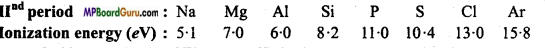Question 39.
Magnitude of Electron affinity increases on moving from left to right in a period. Explain.
On moving from left to right in a period, nuclear charge increases and atomic radius decreases, due to which attraction of the nucleus for the added electron increases. Therefore, in any period, on moving from left to right electron affinity increases.

Question 40.
Explain the periodicity of metallic character in the periodic table.
Elemeiits which lose electrons are electropositive elements and which gain electrons are called electronegative elements. Electropositive elements represent metallic nature whereas electronegative elements represent non-metallic nature. On moving from top to bottom in a group metallic nature increases but in any period on moving from left to right metallic nature decreases.

Question 41.
Of the following pair N and O, whose electron gain enthalpy is more?
Electron gain enthalpy of O is more than N because the valence shell of N -1s2,2s22p3 is half filled, thus it is stable. Thus, tendency to gain an extra e is more in O than in N. Apart from this size of O is smaller than N, thus due to high nuclear charge e is added easily.

Classification of Elements and Periodicity in Properties Class 11 Important Questions Short Answer Type

Question 1.
What do you think? Second electron gain enthalpy like first electron gain enthalpy will be positive, negative or less negative Justify your answer.
O(g)+ e → O;
Δeg H = -141 kJ mol -1
O,sup>-(g) + e → O2-;
Δeg H = +780 kJ mol-1

When oxygen atom gains one electron, to form O ion energy is released. Thus, first electron gain enthalpy of oxygen is negative.
But, when O ion gains one more electron to form O-2 ion, it feels strong electrostatic repulsion. Thus, for the addition of second electron, energy is to be absorbed. Therefore, second electron gain enthalpy of oxygen is positive.

Question 2.
Electron gain enthalpy of fluorine is less negative as compared to chlorine. Give reason.
Electron gain enthalpy (Electron affinity) of fluorine is less negative than chlo¬rine because when one electron enters F, then it enters into a small size (n = 2) energy level where it feels the repulsion of the other electrons present in this level. In Cl electron enters into a larger (n = 3) energy level where it feels very less repulsion force of other electrons present in it.Question 3.
Long form of the periodic table is more superior than Mendeleev’s table. Why?
Long form of periodic table is more superior than Mendeleev’s table because of following reasons:

• Long form of periodic table is based on atomic number which is more fundamental property of an atom.
• Position of every element is decided on the basis of its electronic configuration.
• It represents the similarities in chemical properties, dissimilarities and gradual change in properties of various elements more clearly.
• Here classification of elements is more scientific and reasonable.

Question 4.
Write the main characteristics of Modern periodic table.
The main characteristics of Modem periodic table are :

• This periodic table is based on the electronic configuration of elements.
• Metals and non-metals are placed separately.
• Transition elements are separated from normal elements.
• On the basis of filling of electrons in elements, all the elements are divided into s,p, d and f block.
• Rare earth elements are placed in a suitable place outside, below the main periodic table.

Question 5.
Atomic number of an element is 17. Locate its position in the periodic table giving its electronic configuration.
Electronic configuration of element of atomic number 17 =1s2,2s2 2p6,3s2 3p5

• Electronic configuration of outermost shell is 3s2 3p5. So it is element of p-block.
• Group: If a shell is incomplete, then the number of electrons represents the group of that element. Therefore, it is an element of 7th group.
• Sub-group: If last electron enters into s or p sub-shell, then it is the element of sub-group A.
• Period: Number of shells represents the period.This element is the member of third period.
1. Group = 7
2. Sub-group = A
3. Period = 3

Question 6.
Electronic configuration of an element is 1s2,2s2 2p6,3s2 3p6. In which group this element will be placed and why? Explain.
Electronic configuration of outermost orbit of this element is 3s2 3p6. It is well known that electronic configuration of outermost orbit ns2 np b is of noble gases (except He). Therefore, this element is also a noble gas which belongs to group 18 in modem periodic table.

Question 7.
All transition elements are d -block elements but all d -block elements are not transition elements. Explain.
Elements in which last electron enters into rf-block orbital are called if-block elements or transition elements. General outer electronic configuration of these elements is (n – 1)d1-10, ns0 – 2Electronic configuration of Zn, Cd and Hg is (n-l)d1-10, ns2 but they do not show the characteristics of transition elements.

The d-orbitals of these elements is completely filled in their ground state or in common oxidation state. Therefore, they could not be considered as transition elements. Thus, on the basis of properties all transition elements are d-block elements and on the basis of electronic configuration, all d-block elements are not transition elements.

Question 8.
What do you understand by iso-electronic species? Write the name of such a species which is iso-electronic with the following atoms and ions :
(i) F
(ii) Ar
(iii) Mg2+
(iv) Rb+.
In iso-electronic species number of electrons are same but nuclear charge is different.
In these species, with the increase in nuclear charge, size decreases.
(i) No. of electrons in F = 9 + 1 = 10
(ii) No. of electrons in Ar = 18
(iii) No. of electrons in Mg2+ = 12-2=10
(iv) No. of electrons in Rb+ = 37-1 =36
N3-, O2-, Ne, Na+ and Al3+ species are iso-electronic species of F and Mg2+, P3-, s2, Cl, K+ and Ca2+ species are iso-electronic species of Ar. Similarly, Br Kr and Sr2+ species are iso-electronic species of Rb+.

Question 9.
Among the following pairs whose ionization energy is low and why?
(i) Cl or F
(ii) Cl or S
(iii) K or Ar
(iv) Kr or Xe.
(i) In Cl and F, ionization energy of chlorine is less because size of Cl is bigger than F.

(ii) In Cl and S, ionization energy of S is less because both have same number of shells but nuclear charge of S is less than Cl. Therefore, size of S is bigger than Cl.

(iii) In K and Ar, ionization energy of K is less. There is only 1 electron in the valence shell of K which it can lose easily and form K+ ion whereas due to complete octet in Ar, it is stable and more energy is required to remove an electron.

(iv) In Kr and Xe, ionization energy of Xe is less because size of Xe is bigger than Kr.

Question 10.
On the basis of electronic configuration, how many types of elements are there? Give two examples of each. Or, Explain the various types of elements on the basis of Electronic configuration.
Types of elements on the basis of electronic configuration :
(i) Noble Gas: They are placed in 18th group. Their outermost shell is stable whose configuration is ns2 or ns2p6. Example He, Ne.

(ii) Representative elements or s and p-block element: Elements of group 1,2 and 13 to 17 are called normal or Representative elements. Their outermost shell is incomplete and general electronic configuration of outermost shell is ns1 to ns2p5.
It includes s-and p-block. (s-block element Na, Mg and p-block element Cl, Br)

(iii) Transition element or d-block elements: It includes elements of group 3 to 12. Their outer two shells are incomplete. Elements of group 3-12 which are situated in the middle of the periodic table are called Transition elements. General Electronic configuration of outermost shell is (n – 1) d1-10 ns1-2
Example: Cr, Cu.

(iv) Inner Transition Elements or f-block Element: Their outer three shells are incomplete. These are known as /-block or rare earth elements. It includs Lanthanides and Actinides. These are mainly situated at the bottom of the periodic table. General electronic configuration of its outermost shell is (n – 1)f1-14 (n-1) d0-1 ns2
Example: Lanthanide: Cerium (Ce), Praseodymium (Pr)
Actinide: Thorium (Th), Uranium (U).Question 11.
What are s-block elements? Write their main properties.
In their electronic configuration, last electron enters into s sub-shell and only outermost shell is incomplete, s sub-shell of outermost shell contains 1 or 2 electrons and their general electronic configuration is ns1 or 2.

Main properties of s-block :

• In the outermost orbit of element, there are 1 or 2 electrons.
• The electronic configuration of its outer orbit is nslor2.
• The oxidation state of it is +1 or +2.
• Except He and H all elements are metals. In 1st group the elements are alkaline elements and in II nd group, the elements are alkaline earth elements.
• These form ionic compounds.
• These are strong reducing agents.
• The oxides of these are alkaline.
• These react with water and acid and give out hydrogen.

Question 12.
What are the p-block elements? What are their main characteristics?
In the electronic configuration the last electron enters in top sub-shell, it is known as p -block element. In the outermost shell, there are 2 electrons in s sub-shell and 1 to 6 electrons in p sub-shell configuration of their valence shell is ns2 np1-6

General characteristic of p-block elements :

• These elements have 2 electrons in s sub-shell and 1 to 6 electrons in p sub-shell in outermost orbit. Only in zero group elements, outer shell is completed. ‘
• Definite positive or negative oxidation numbers are represented by the elements. Some of the elements show variable valencies.
• These elements form simple ions as well as complex ions of CO32-, NO3.
• These are generally non-metals and metalloids. Some of the elements are heavy elements, e.g., Pb, Bi, etc.
• Oxides are acidic in nature. Some oxides are amphoteric, e.g., PbO, SnO etc.
• These elements form covalent compounds with each other but with the elements of 5-block form electrovalent compounds.

Question 13.
Electronic configuration ofthe element is 1s2,2s2 2p6,3s2 3p6,4s2and its mass number is 40. State the total number of neutrons in the nucleus and also deduce its position and name of the element in the periodic table.
Number of electrons in the element = 20
Number of protons = 20
Mass number = Number of protons + Number of neutrons
40 = 20 + Number of neutrons
∴ Number of neutrons = 20
Atomic number of that element is 20 and element is Calcium (Ca) which belongs to period 4 and group 2.

Question 14.
What is meant by Electronegativity of an element? How is it different from electron affinity? How does Electronegativity change in the periodic table?
Electronegativity: The property of attracting the shared pair of electrons between two atoms in a compound towards itself is called electronegativity.

Electron affinity: The energy released due to entrance of an electron in outermost shell of an atom in gaseous state is called electron affinity. value of first electron affinity of an element is negative because this process is exothermic, whereas in second eleciron affinity energy is absorbed because negatively charged ion repels the incoming electron.

Difference: Electron affinity is the property of binding the extra electron whereas electro-negativity is the capacity of attracting electron by an atom of a molecule.

Gradation: On moving from left to right, due to decrease in atomic size and increase in nuclear charge electro-negativity increases. On moving from top to bottom in a group, with the increase in atomic size electronegativity decreases

Question 15.
Write uses of Mendeleev’s periodic table.
Following are the importance or uses of Mendeleev’s periodic table :
(i) Helpful in the study of Elements: In the study of properties of elements in the periodic table.

(ii) Elements of same group possess similar properties, so by studying the general properties of the group, properties of all the elements can be known.

(iii) In finding of atomic mass: From the position of elements in the periodic table its valency can be known. If the equivalent mass of that element is known, its atomic mass can be known.
Atomic mass = Equivalent mass × Valency

(iv) Helpful in discovery of new elements: At the time of preparation of periodic table, Mendeleev had left many places for new elements but the properties of these elements were categorized by him. After discovery of these new elements, it is seen that the properties are exactly same as predicted by Mendeleev.

(v) Helpful in research: Elements and their compounds are studied comparatively with the help of periodic table. So, periodic table is helpful in research.Question 16.
Where are Transition elements placed in the periodic table? Or, What are transition elements ? Explain four main properties of transition elements.
Transition elements: The elements which are in between s-block and p-block are called as transition elements or elements in which d-orbitals are partially filled are called transition elements. In this, last electron goes to d-orbital of orbit inner to outer so called d- block elements. Boiling point, melting point and densities of these elements are high.
Example: Cr, Mn, Fe, Cu, etc.

Properties :

• In these two outer orbits are incomplete,
• Electronic configuration is (n – 1) d1-10 ns1 or 2,
• It has metallic properties,
• These elements show variable oxidation state,
• These form coloured ions,
• These form complex salt,
• These are good catalysts,
• These form complex compounds,
• These are generally diamagnetic and
• These also form with non-metal compounds.

Question 17.
What are Inner transition elements? Write general characteristic of inner transition elements.
Three outer shells of these elements are incomplete. In their electronic configuration, the last electron enters into the sub-shell of the third inner (anti penultimate) shell. Therefore, they are known as d-block elements. Both the series of Lanthanides and Actinides elements are the elements which join group 3 and group 4, therefore they are known as inner transition elements and their general electronic configuration is ns2 f1-14 (n-1)d0-1 ns2
Lanthanides: In their electronic configuration, last electron enters 4/sub-shell.
Actinides: In their electronic configuration, last electron enters 5/sub-shell.

General characteristic of Inner Transition Elements :

• Electron enters in/sub shell of (n – 2) shell.
• They exhibit variable oxidation state.
• They all show metallic nature.
• Their ions are generally coloured.
• They form complex ions.
• Form electrovalent compounds.

Question 18.
Define modern periodic law. Discuss briefly the periodic table on the basis of this law.
On the basis of modem periodic table, Bohr gave a systematic arranged periodic table which is called long form of periodic table. The physical and chemical properties of elements are periodic function of their atomic number.

Periods: In modern periodic table, there are also seven horizontal rows which are known as periods.

• First period is very short period which contains only two elements.
• Second and third periods are short period which contain 8-8 elements.
• Fourth and fifth periods have 18-18 elements.
• In sixth period total 32 elements are present. From atomic number 58 to 71 are 14 lanthanides which are placed separately below the periodic table.
• Seventh period is incomplete which contains 21 elements. From atomic number 90 to 103, total 14 actinides are also included which are placed separately.
• Period number shows the principal quantum number of outer shell.
• Outer orbit of each period start with ns and ends with ns , np configuration.
• Except first period, all period start with alkali metal and finishes at inert gases.
• 14 lanthanides and 14 actinides in two separate series are placed out-side of the periodic table.

Groups: There is 18 vertical columns in the modem periodic table which are called groups. These groups are expressed by numbers 1, 2, 3,…………….18.
1. All the elements of same group have similar electronic configuration in outermost shell.
2. On the basis of electronic configuration elements are classified into 4 blocks :

• s-block elements,
• p-block elements,
• d-block elements,
• f – block elements.

Question 19.
Compare modern periodic table with Mendeleev’s periodic table (Any 3 points).
Comparison between Modern periodic table and Mendeleev’s periodic table

 Modern periodic table Mendeleev’s periodic table 1. In modern periodic table elements are arranged in the increasing order of their atomic numbers. In Mendeleev’s periodic table elements are arranged in the increasing order of their atomic masses. 2. In modern periodic table there are 18 vertical columns called groups. In Mendeleev’s periodic table there are 9 vertical columns called groups. 3. In modern periodic table at the end of every period, there is inert gas. In Mendeleev’s periodic table zero group is added later. 4. In this, elements with dissimilar properties are placed separately. In this elements with different properties are placed in one group. 5. Isotopes of all elements possess same atomic number, so separate places are not required for them. In Mendeleev’s periodic table each iso-tope should be alloted separate place, but there was no separate place for isotopes.

Question 20.
How does atomic size change with the increase in atomic number?
In group: In a group on moving below, atomic size increases because electron enters in new orbit (shell). Along with increase in number of shells, effective nuclear charge also increases but increase in number of shells dominates over nuclear charge.

In period: In a period on moving from left to right atomic size decreases because electrons enter same shell and effective nuclear charge increases, which reduce the size.

Question 21.
What changes occur in the reducing and oxidizing properties of elements in periodic table ?
Reducing property: Elements which loose electrons to form positive ions are called reducing agents. Reducing nature of any element depend on the nature of an element to loose its electron. Due to larger size of alkali metals, their ionization potential is low.

Therefore, they can easily loose electrons, thus alkali metals are strong reducing agents. On moving from left to right in a period reducing power decreases and on moving from top to bottom, reducing power increases.

Oxidizing property: Elements which gain electron act like oxidizing agents. Oxidiz¬ing nature of an element depends on its tendency to gain electron. Halogens can easily accept electrons therefore, they are strong oxidizing agents. On moving from left to right in a period. Oxidizing power increases and on moving from top to bottom in a group oxidizing power decreases.Question 22.
Explain Ionization enthalpy (energy) and Electron affinity.
Ionization enthalpy: The amount of energy which is required to separate the outermost electron from atom is called ionic energy or ionic potential i.e., to convert atom into positive ion the necessary energy required is called as ionization energy. Its unit is kJ mol-1.

So, M(g) + Energy → M(g)+e ( ΔH = + ve)

Electron affinity: The electron affinity of an atom is the energy released, when an electron is added to a neutral atom. It is expressed in kcal/mol. Electronegativity is a relative number on an arbitrary scale while electron affinity is expressed in the units of energy (kcal/ mol).

This way in the addition of an electron to neutral isolated atom energy is released and electron affinity is positive, but in an anion energy is required to add an electron because the anion opposes the entrance of an electron. Thus, electron affinity for charged ions is negative.

Question 23.
Atomic radius: Atomic size is a very important property of atom, because it is related to various other physical and chemical properties. For the study of atomic size, atom is considered to be spherical. Thus, its radius determines its size.
“Normally the distance between the centre of nucleus, to the outermost shell of an atom is called Atomic radius.”

Ionic radius: The distance of the affected region from the nucleus to the electron cloud is called ionic radius or in an ionic crystal half the distance of the nuclei between two ions is called ionic radius of those ions.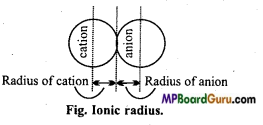Classification of Elements and Periodicity in Properties Class 11 Important Questions Long Answer Type

Question 1.
What is the significance of the terms: ‘Isolated gaseous atom’ and ‘ground state’ while defining the Ionization enthalpy and Electron gain enthalpy.
Ionization enthalpy is the minimum energy required to remove the outermost electron from an isolated gaseous atom (X).
X(g) → X+(g) + e

This force by which electron is attracted by the nucleus, by other atoms present in the molecule or is also affected by other neighbouring atoms. Thus, ionization enthalpy is always determined in gaseous state because in gaseous state intermolecular space is maximum and intermolecular attractive force is minimum. Again, ionization enthalpy is measured at low pressure, because it is not possible to isolate an atom but by lowering the pressure intermolecular force can be reduced. That is why, in the definition of Ionization enthalpy, isolated gaseous atom is added in ground state.
Electron affinity is the energy released when an electron is gained by an isolated gas¬eous atom (X) in ground state to form an anion.
X+(g) + e →X(g)

Most stable state of an atom is ground state. If isolated gaseous atom is in excited state then on gaining an electron comparatively less energy is released. Thus, electron gain en¬thalpy of gaseous atoms is always measured in ground state. Thus, ‘ground state’ and isolated gaseous atom should always be included in the definition of electron gain enthalpy.

Question 2.
In the following pairs of elements which element has more negative electron affinity :
(i) O or F,
(ii) F or Cl.
(i) Oxygen and Fluorine both are situated in second period. On moving from left to right in a period electron gain enthalpy goes on becoming more negative. On moving from oxygen to fluorine, along with the increase in atomic number, value of effective nuclear charge increases and size of atom goes on decreasing, due to which attractive force by the nucleus for the incoming electron increases. That is why, electron gain enthalpy of Fluorine is comparatively more negative than the electron gain enthalpy of oxygen. Also Fluorine gains one electron and achieves stable configuration.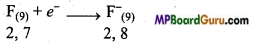Thus, electron gain enthalpy of Fluorine (-328 kJ mol-1) is more negative than oxygen (-141 kJ mol-1).
(ii) On moving down in a group, negativity of electron gain enthalpy gradually decreases. But, electron gain enthalpy of Chlorine (-349 kJ mol-1) is more negative than the electron gain enthalpy of Fluorine (-328 kJ mol-1). This is due to small size of Fluorine compared to 3p-orbital (Cl), electron-electron repulsion in 2p-orbital (F) is more. Thus, the incoming electron feels more repulsion in Fluorine as compared to Chlorine. That is why, electron gain enthalpy of Chlorine is more negative than Fluorine.Question 3.
What are the main defects of Mendeleev’s periodic table? How can they be removed by modern periodic table?
Defects of Mendeleev’s periodic table :

• Position of hydrogen is indefinite. ,
• Heavier elements were placed before lighter elements.
• Elements with dissimilar properties were kept in the same group.
• Element of similar properties were kept separately in different groups. ‘
• Position of eighth group elements is not suitable.
• Isotopes were not given place.
• No position was given to inert gases.

In modem periodic table, elements are arranged in the increasing order of atomic number by which various defects of Mendeleev’s table were removed.

1. Elements of higher atomic mass were placed before elements of lower atomic mass : Keeping the elements of higher atomic mass before the elements of lower atomic mass. In modem periodic table elements are kept in the increasing order of atomic number due to which the defects on the basis of atomic mass are removed.
2. Position of isotopes: Isotopes of an element possess same atomic number, therefore there is no necessity of giving them separate position in the periodic table.
3. Elements with different properties have been placed in different positions: In modem periodic tables, elements with different properties are kept separate. Like elements of sub-group IA are placed in group I and elements of sub-group IB Cu, Ag, Au are placed in group II.
4. Position of inert gases: In modem periodic table, inert gases are placed between strongly electronegative elements (group 17) and strongly electropositive elements (group I) which acts as a bridge.

Question 4.
What is ionization energy? Explain the factors affecting ionization energy.
Ionization energy: The amount of energy which is required to separate the outermost electron from atom is called ionic energy or ionic potential i.e., to convert atom into positive ion the necessary energy required is called as ionization energy. Its unit is kJ mol-1.
So, M(g) + Energy → M+(g) + e (ΔH = +ve)
M(g) – Electron → M+(g) + Ionisation energy.
Unit: Unit of ionization energy is kJ/mol

Factors affecting ionization energy :
(i) Size of atom or ion : Greater the size of atom or ion, weaker are the forces of attraction and lower is the value of ionization energy.
(ii) Nuclear charge: Greater the nuclear charge, more is the attraction for electrons and hence, greater is the value of ionization energy.

(iii) Shielding effect: In multielectron atom with increase in atomic number, shielding effect increases due to which valence shell electron feels lesser attraction and hence, value of ionization energy is lower.

(iv) Penetration effect: Closer the orbitals to the nucleus, more is the penetration of it for the nucleus. That is it experience greater attraction. Thus, value of ionization energy is higher, s electron of the same shell is strongly held as compared to p, d,f. Thus, order of ionization energy is s>p> d>f.

(v) Half filled and fully filled orbitals: Completely filled and half-filled orbitals are more stable than any other arrangement. Thus, value of ionization energy is higher for it.

(vi) Configuration of outermost shell: Elements which have 8 electrons in their outermost shell (except He) are more stable and their ionization energy is very high.

(vii) Periodicity:
(a) Periodicity in group: On moving from top to bottom in a group, atomic radius increases due to which less energy is required to remove the electron from the valence shell. Thus, on moving from top to bottom in a group ionization energy decreases.
(b) Periodicity in period: On moving from left to right in a period, due to increase in nuclear charge and decrease in atomic radius, more energy is required to remove an electron from the valence shell. Thus, on moving from left to right in a period value of ionization energy increases.Question 5.
Atomic radius: Atomic radius means size of atom. It can be measured by X-rays or other spectroscopic methods. For non-metals it is also known as covalent radius and for metal elements it is known as metallic radius.

Covalent radius: Half the distance between the nucleus of two atoms bonded by a covalent bond in a molecule is called covalent radius. For example, for Chlorine molecule bond distance is 198pm, thus half of this, 99pm is the covalent radius or atomic radius of Chlorine atom.

Ionic radius: Ionic radius means size of ion (cation or anion). It can be measured by measuring the distance between the cation and anion in an ionic crystal. A cation is always smaller than its parent atom because due to removal of one or more electron, effective nuclear charge increases. Anion is always larger than the present atom because due to gain of electron value of effective nuclear charge decreases. For example, Ionic radius of Na+ ion (95pm) is less than atomic radius of Na atom (186pm) whereas ionic radius of F (136pm) is more than atomic radius of F (72pm).

Question 6.
What do you understand by electron affinity? Explain the factors affecting it
Electron affinity: The electron affinity of an atom is the energy released, when an electron is added to a neutral atom. It is expressed in kcal/mol. Electronegativity is a relative number on an arbitrary scale while electron affinity is expressed in the units of energy (kcal/mol).

A(g)+e → A(g) + E1 (Exothermic)
A(g) + e → A2- – E2 (Exothermic)
A2-(g) + e → A3-(g) – E3(Exothermic)

This way in the addition of an electron to neutral isolated atom energy is released and electron affinity is positive, but in an anion energy is required to add an electron because the anion opposes the intrance of an electron. Thus, electron affinity for charged ions is negative.

Factors affecting electron gain enthalpy: Some important factors affecting electron gain enthalpy are:
(i) Atomic size: As the atomic size increases, the distance between the nucleus and the incoming electron increases. This results in lesser attraction. Consequently, electron gain enthalpy becomes less negative.

(ii) Effective nuclear charge: Greater the nuclear charge, greater will be attraction for the incoming electron and as a result, Δeg H becomes more negative.

(iii) Outermost electronic configuration: Atoms having stable electronic configuration have lesser tendency to accept the electron. Due to this the value of electron gain enthalpy becomes less iiegative.

(iv) Half filled and fully filled orbitals: Half filled and fully filled orbitals are more stable and does not easily accept an electron in this state. Therefore, electron affinity of such elements is zero.

(v) Periodicity :
(a) Periodicity in period: In a period, on moving from left to right, value of electron affinity generally increases because nuclear charge increases and atomic size decreases. As a result, attraction of nucleus for the accepted electron increases.
(b) Periodicity in group: On moving from top to bottom in a group; due to increase
in atomic size attractive force between the nucleus and incoming electron decreases, due to which electron affinity decreases.

Question 7.
In periodic table on moving left to right, what change in following proper-ties occurs:
(ii) Electronegativity,
(iii) Ionization potential,
(iv) Metallic character,
(v) Valency.
(i) Atomic radii: On moving from left to right in the period, the atomic radii decreases because electron enter in same shell and due to increase in nuclear charge atomic size shrinks. For example, in 3rd period, Na atom has largest size while Cl atom is shortest.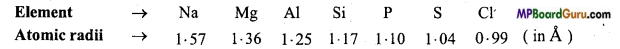(ii) Electronegativity: In a period on moving towards right side electronegativity increases due to decrease in atomic size and increase in nuclear charge.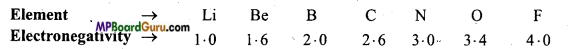(iii) Ionisation potential (I.P.): When we move in a period from left to right ionisation potential of atoms also increases. It is due .to increase in nuclear charge and decrease in atomic size.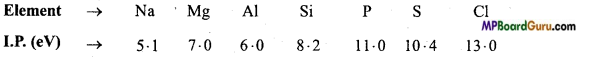(iv) Metallic character: Metallic character decreases on moving from left to right in a period.
(v) Valency: On moving left to right in a period, valency of element with respect to oxygen increases from 1 to 7 while in respect of hydrogen or chlorine it increases from 1 to 4 and then reduces up to 1.

Question 8.
The first (ΔiH1) and the. second (ΔiH2) ionization enthalpies (in kJ mol-1) and (ΔegH) electron gain enthalpy (in kJ mol-1) of a few elements are given below :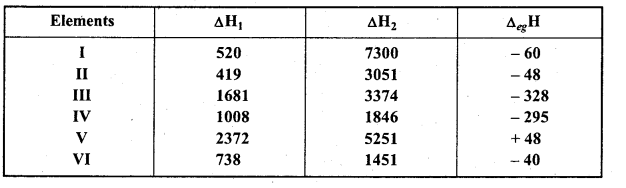Which of the above elements is likely to be :

• The least reactive element
• The most reactive metal
• The most reactive non-metal
• The least reactive non-metal
• The metal which can form stable binary halide of the formula MX2 (X = halogen).Question 9.
Which metal is alkali and which forms stable covalent compound.
(i) The least reactive element is (V) because its first ionization enthalpy is maximum and electron gain enthalpy is positive. Electron gain enthalpy of inert gas element is positive. Values given for element (V) corresponds to He.

(ii) Most reactive metal is element (II). Its first ionization enthalpy is least and electron gain enthalpy is less negative. The values given for element (II) corresponds to K.

(iii) Most reactive non-metal is (III) because its first ionization enthalpy is highest and electron gain enthalpy is maximum negative. Values given for element (III) corresponding to F.

(iv) Least reactive non-metal is (IV) because its first ionization enthalpy is not high but electron gain enthalpy is more negative. Values given for element (IV) corresponds to I.

(v) Metal which forms stable binary halide (MX2) is (VI). Element (VI) is alkaline earth metal because its first ionization enthalpy is less but is more than Alkali metals (II). Secondly, difference between first and second enthalpies is very less. Values given for element (VI) corresponds to Mg.

(vi) First ionization enthalpy of element (I) is low but second is high. Its electron gain enthalpy is less negative. Thus element (I) is alkali metal. Values given for element (I) corresponds to Li. Li predominantly forms stable covalent compound of formula MX.

Classification of Elements and Periodicity in Properties Class 11 Important Questions Objective Type

Question 1.
Which of the following electronic structures is of a metal:
(a) 2, 8, 8
(b) 2, 7
(c) 3, 8, 2
(d) 2,8,4.
(c) 3, 8, 2

Question 2.
Which of the following has the highest ionization potential:
(a) Na
(b) Mg
(c) C
(d) F.
(d) F.

Question 3.
Which of the following is most electronegative :
(a) Oxygen
(b) Chlorine
(c) Nitrogen
(d) Fluorine.
(d) Fluorine.

Question 4.
With increase in atomic mass in a period in periodic table there is :
(a) Increase in property of electropositivity
(b) Increase in property of electronegativity
(c) Increase in chemical activity
(d) Decrease in chemical activity.
(b) Increase in property of electronegativity

Question 5.
Of the following, whose ionic radii is largest:
(a) K+
(b) O2+
(c) B2+
(d) CT.
(a) K+

Question 6.
Which of the following is the electronic configuration in last orbit of a noble gas:
(a) s2p3
(b) s2p4
(c) s2p5
(d) s2p6.
(d) s2p6.

Question 7.
Those elements in which s and p orbitals are complete :
(a) Normal elements
(b) Transition elements
(c) Inert gas
(d) Halogens.
(c) Inert gasQuestion 8.
Which of the following has bigger size :
(a) Br
(b) F
(c) I
(d) I.
(d) I.

Question 9.
Which of the following is of smallest size :
(a) Al
(b) Al+
(c) Al2+
(d) Al3+.
(d) Al3+.

Question 10.
Which of the following is a strong reducing agent:
(a) F
(b) Cr
(c) Br
(d) I.
(d) I.

Question 11.
The element having highest value of first ionization potential:
(a) Boron
(b) Carbon
(c) Nitrogen
(d) Oxygen.
(c) Nitrogen

Question 12.
Which of the following has highest value of ionization potential:
(a) Ca
(b) Ba
(c) Sr
(d) Mg
(d) Mg

Question 13.
An element having atomic number 20, will be placed in periodic table in the group:
(a) 4
(b) 3
(c) 2
(d) 1.
(c) 2

Question 14.
Chromium [atomic number = 24] is a :
(a) Transition element
(b) Inert gas element
(c) Normal element
(d) Inner transition element.
(a) Transition elementQuestion 15.
Ionization potential of elements in a group of periodic table:
(a) Increases with atomic size
(b) Decreases with increase in atomic size
(c) Remains constant
(d) Changes irregularly.
(b) Decreases with increase in atomic size

Question 16.
Metallic character on moving from left to right in a periodic table :
(a) Increases
(b) Decreases
(c) Remains constant
(d) First decreases then increases.
(b) Decreases

2. Fill in the blanks:

1. Electron gain enthalpy of noble gases is …………….. .
zero

2. Electron gain enthalpy of Be and Mg in second group is ……………… .
zero

3. On moving from left to right in a period ionization energy …………….. .
increases

4. Second ionization potential of any element is always …………….. than the first ionization potential.
higher

5. Most electronegative element is placed in …………….. group of the periodic table.
VII A

6. Similarity in properties of Li and Mg, and Be and A1 is due to …………….. relationship.
diagonal

7. In modem peiodic table, there are total …………….. columns and …………….. periods.
18,7

8. With the increase in nuclear charge, atomic radius in a period …………….. .
decreases

9. Electron affinity of chlorine is …………….. than fluorine.
more

10. Newland stated that if elements are arranged in increasing order of elements then every …………….. element which show properties similar to the first element.
eighth.3. Match the following:

 ‘A’ ‘B’ 1. Which is a noble gas (a) Carbon 2. Which is a halogen (b) Potassium 3. Which is an alkali metal (c) Fluorine 4. Which is the element of valency four. (d) Neon.

1. (d) Neon
2. (c) Fluorine
3. (b) Potassium
4. (a) Carbon.

1. Other name of Eka silicon.
Germanium

2. Number of electrons in N3- and O2- is same. Thus they are called ……… .
Isoelectronic

3. Which is the most stable oxidation state of aluminium?